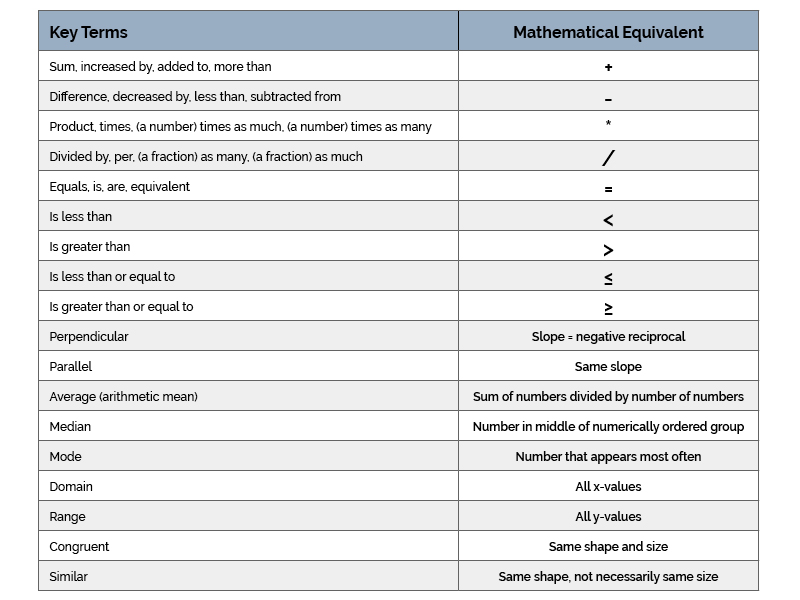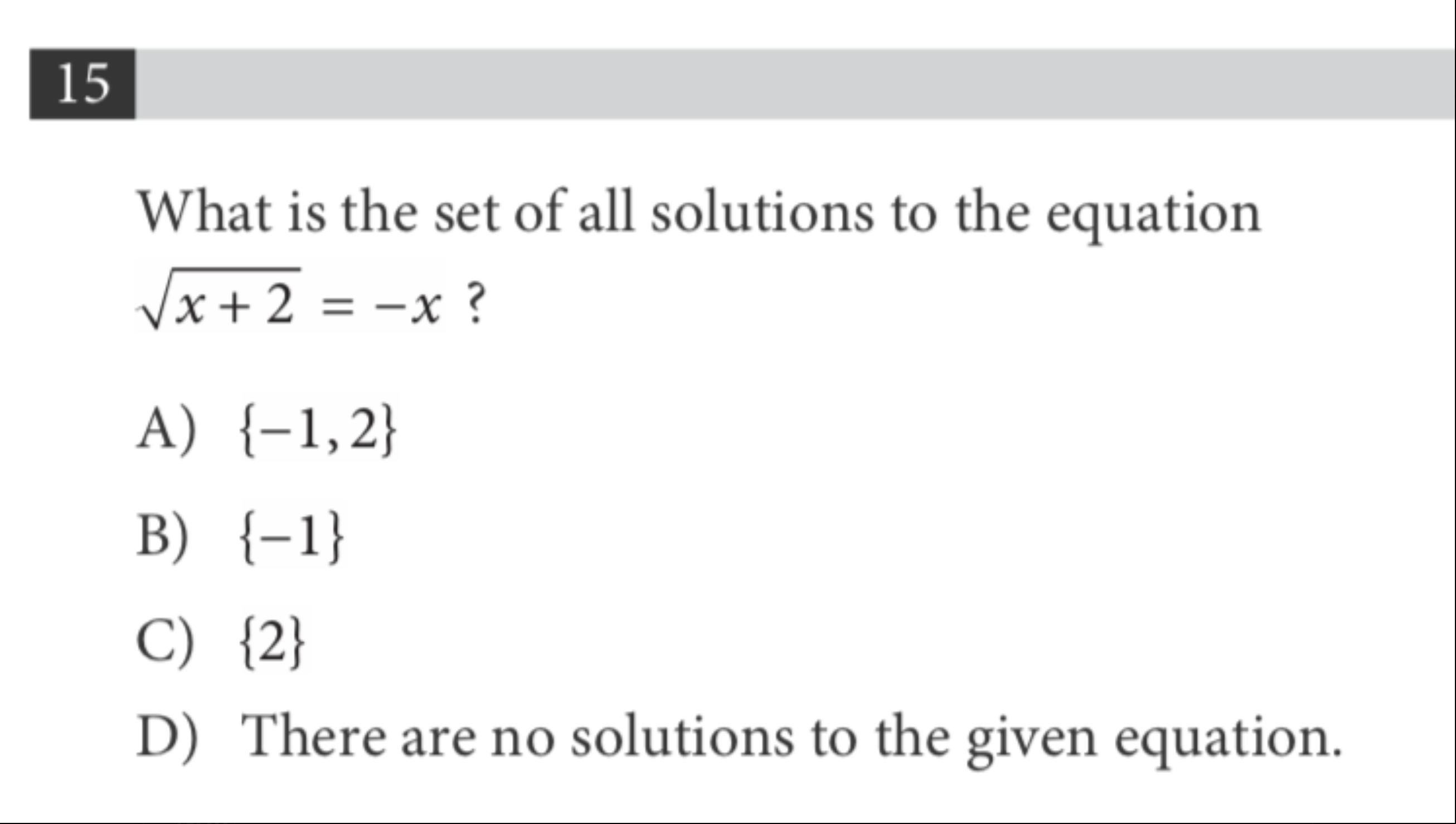Time is Running Out! Get Expert Guidance on Letters of Continued Interest and Application Edits.

a

# Five SAT and ACT Math Strategies

Sep 30, 2020

Some of the most helpful SAT and ACT Math strategies are some of the oldest tricks in the book. Even though many students are familiar with these techniques, we find that they don’t use them as often as they could. Here are some tips that, when used properly, will help you to earn the score you need to get into your dream school.

## Strategy #1: Underline Key Terms

The first step to solving an SAT or ACT math problem is identifying and underlining key information in the question, including key terms and expressions. This will not only help you to engage with the problem and focus on its most important components, but also keep you from making silly mistakes like finding x when the problem is asking you to find y. When underlining, double check to make sure you are identifying the key terms correctly — all of your key terms should be directly related to the topic the question is testing you on.

## Strategy #2: Translate Key Terms into Math Expressions

Once you’ve identified a question’s key terms, you should translate them into their mathematical equivalent and determine the first step to solving the problem. For example, if you underlined two variables and the word “equals” or “is” in a word problem, you should recognize that you are most likely working on a system of equations question. From there, you should set up two equations using the variables and equal signs. If you see the phrase “more than,” you should likely set up an equation with addition, while if you see “less than,” you should likely set up an equation with subtraction. Here is a table to help you to translate key words into mathematical expressions.## Strategy #3: Use Process of Elimination

As soon as you see an answer you know cannot be correct, you should immediately cross it off. This will both prevent you from accidentally choosing it, and help shift your focus to the other answer choices, guiding you towards the right answer. This technique can also boost your confidence — knowing the wrong answer can help you believe in your ability to find the right one.

## Strategy #4: Plug in Possible Answers

After you’ve narrowed down your answer choices, plugging the remaining options into the equation is often the fastest way to solve a problem. Even if you’ve solved a problem using a different method, plugging in answers can be helpful in double checking your work. The question below (from College Board SAT Practice Test #9) shows how useful this technique can be.The fact that there are only two numerical answer options (-1 and 2) gives us the hint that plugging in answers would be the fastest way to solve this problem. To plug in answers, we first need to make sure we understand what the question is asking us to find: the solutions to the equation. This just means that we are looking for the value(s) of x that make the equation true. With that in mind, we can choose one of the answers and plug its values into the original equation.

√(-1+2)=1=-(-1)

Clearly, this is true.

Now let’s try 2.

√(2+2)=2=-2

Clearly, this is not true.

Based on plugging in these two numbers, we can see that answer choice B is the correct answer. To become more comfortable with this strategy, you could go through SAT or ACT practice tests, identify which problems could be solved by plugging in answers, and then solve them. Practicing this will help you quickly recognize which questions you can solve using this technique on test day.

## Strategy #5: Dissect Challenging Questions

It is very rare for you to receive a test that isn’t difficult in some way. Standardized test makers do this on purpose to see what you do when you face a challenge. They also do this to see how you compare to the rest of the pool of test takers. Whenever you face a challenging question, instead of giving up, pretend you are a detective and piece the relevant information together, as we did in the previous example. Keep in mind that wrong answers aren’t penalized on the SAT or ACT, so it’s always worth giving every question a shot!

The SAT and ACT Math sections are notoriously tricky, but practicing these five strategies can help you feel calm, confident, and prepared on test day.

Contents